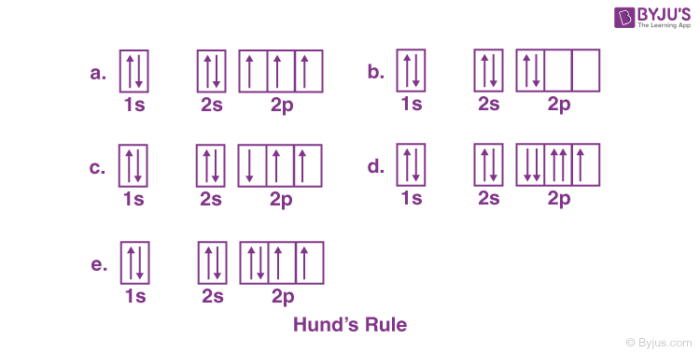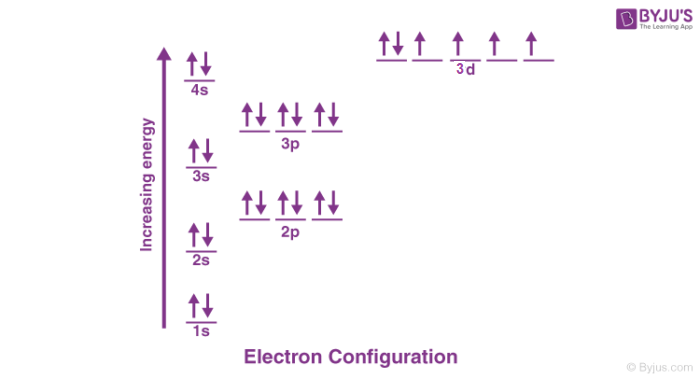# Hund's Rule

## Introduction to Hund’s Rule

Aufbau principle tells us that the lowest energy orbitals get filled by electrons first. After the lower energy orbitals are filled, the electrons move on to higher energy orbitals. The problem with this rule is that it does not tell about the three 2p orbitals and the order that they will be filled in.

According to Hund’s rule:

• Before the double occupation of any orbital, every orbital in the sub level is singly occupied.
• For the maximization of total spin, all electrons in a single occupancy orbital have the same spin.

An electron will not pair with another electron in a half-filled orbital as it has the ability to fill all its orbitals with similar energy. Many unpaired electrons are present in atoms which are at the ground state. If two electrons come in contact they would show the same behaviour as two magnets do. The electrons first try to get as far away from each other as possible before they have to pair up.## Hunds Rule of Maximum Multiplicity

Hunds Rule of Maximum Multiplicity rule states that for a given electron configuration, the term with maximum multiplicity falls lowest in energy. According to this rule electron pairing in p, d and f orbitals cannot occur until each orbital of a given subshell contains one electron each or is singly occupied.

## State Hund’s Rule

It states that:

1. In a sublevel, each orbital is singly occupied before it is doubly occupied.

2. The electrons present in singly occupied orbitals possess identical spin.

## Explanation of Hund’s Rule

The electrons enter an empty orbital before pairing up. The electrons repel each other as they are negatively charged. The electrons do not share orbitals to reduce repulsion.

When we consider the second rule, the spins of unpaired electrons in singly occupied orbitals are the same. The initial electrons spin in the sub-level decides what the spin of the other electrons would be. For instance, a carbon atom’s electron configuration would be 1s22s22p2. The same orbital will be occupied by the two 2s electrons although different orbitals will be occupied by the two 2p electrons in reference to Hund’s rule.

## Electron Configuration and its PurposeThe above image helps in understanding the electronic configuration and its purpose. The valence shells of two atoms that come in contact with each other will interact first. When valence shells are not full then the atom is the least stable. The chemical characteristics of an element are largely dependent on the valence electrons. Similar chemical characteristics can be seen in elements that have similar valence numbers.

The stability can also be predicted by the electron configuration. When all the orbitals of an atom are full it is most stable. The orbitals that have full energy level are the most stable, for example, noble gases. These type of elements do not react with other elements.

## Hund’s rule of maximum multiplicity

The rule states that, for a stated electron configuration, the greatest value of spin multiplicity has the lowest energy term. It says if two or more than two orbitals having the same amount of energy are unoccupied then the electrons will start occupying them individually before they fill them in pairs. It is a rule which depends on the observation of atomic spectra, which is helpful in predicting the ground state of a molecule or an atom with one or more than one open electronic shells. This rule was discovered in the year 1925 by Friedrich Hund.

## Uses of Hund’s Rule:

It has wide applications in the following –

It is majorly used in atomic chemistry, quantum chemistry, and spectroscopy, etc.

### What type of configurations violate Hund’s rule?

Every orbital of the same energy must have at least one electron which has identical spin before you deposit two in the same orbital.

### Briefly explain the working of Hund’s rule.

As per its first rule, electrons before pairing up always enter an empty orbital. Since electrons are negatively charged particles they repel each other. When they occupy their orbitals they can easily minimize repulsion.

### State Aufbau principle.

It states that in an atom’s ground state the electron enters the lowest energy orbital first and later the higher energy orbitals.